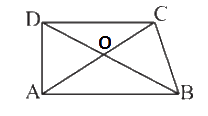# Ex.6.4 Q5 The-Triangle-and-its-Properties Solutions - NCERT Maths Class 7

Go back to  'Ex.6.4'

## Question

$$ABCD$$ is quadrilateral. Is $$AB \!+\! BC \!+\! CD \!+\! DA\! <\! 2 (AC \!+\! BD)?$$Video Solution
Triangle & Its Properties
Ex 6.4 | Question 5

## Text Solution

What is known?

$$ABCD$$ is a quadrilateral. $$DB$$ and $$AC$$ are diagonals.

What is unknown?

Is $$AB \!+\! BC \!+\! CD \!+\! DA \!<\! 2 (AC \!+\! BD)?$$

Reasoning:

In this question, it is asked to check Is $$AB \!+\! BC \!+\! CD \!+\! DA \!<\!2(AC \!+\! BD)$$ or not.
This question is based on the property that the sum of lengths of two sides of a triangle is always greater than the third side.$$“O”$$ is the centre of the quadrilateral.  Now visually identify that the quadrilateral $$ABCD$$ is divided by diagonals $$AC$$ and $$BD$$ into four triangles. Now, take each triangle separately i.e. triangle $$AOB, COD, BOC$$ and $$AOD$$ and apply the above property and then add $$L.H.S$$ and $$R.H.S$$ of the equation formed.

Steps:

In triangle $$AOB,$$

$$AB < OA + OB{\rm{ }} \qquad \ldots \ldots .{\rm{ }}\left( 1 \right)$$

In triangle $$COD,$$

$$CD < OC + OD{\rm{ }} \qquad \ldots \ldots .{\rm{ }}\left( 2 \right)$$

In triangle $$AOD,$$

$$DA < OD + OA{\rm{ }} \qquad \ldots \ldots .{\rm{ }}\left( 3 \right)$$

In triangle $$COB,$$

$$BC < OC + OB{\rm{ }} \qquad \ldots \ldots .{\rm{ }}\left( 4 \right)$$

Adding equation $$(1),$$ $$(2),$$ $$(3)$$ and $$(4)$$ we get,

\begin{align}& \left[ \begin{array}& AB\!+\!BC\!+\!\\CD\!+\!DA \end{array} \right]\!<\!\left[ \begin{array}& OA\!+\!OB\!+\! \\ OC\!+\!OD\!+\!\\OD\!+\!OA\!+\! \\ OC\!+\!OB \\ \end{array} \right] \\ =& \left[ \begin{array}& AB\!+\!BC\!+\!\\CD\!+\!DA\end{array} \right]<\left[ \begin{array}&2OA\!+\!2OB\!+\!\\2OC\!+\!2OD\end{array} \right] \\ =& \left[ \begin{array} & AB\!+\!BC\!+\! \\ CD\!+\!DA \\ \end{array} \right]<\left[ \begin{array}&2(OA\!+\!OB)\!+\!\\2(OC\!+\!OD)\end{array} \right] \\ = & AB\!+\!BC\!+\!CD\!+\!DA\!<\!2(AC\!+\!BD) \\ \end{align}

Yes, it is true.

Useful Tip

Whenever you encounter problems of this kind, it is best to think of the property based on sum of lengths of any two sides of a triangle is always greater than the third side.

Learn from the best math teachers and top your exams

• Live one on one classroom and doubt clearing
• Practice worksheets in and after class for conceptual clarity
• Personalized curriculum to keep up with school